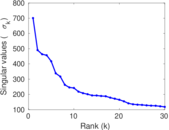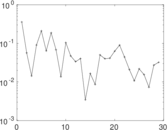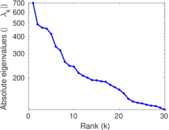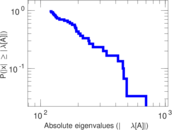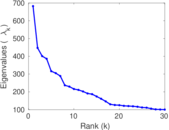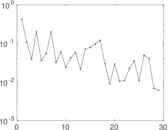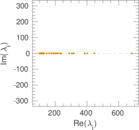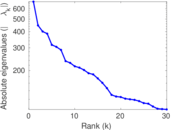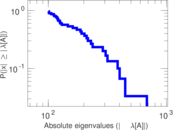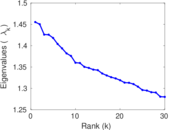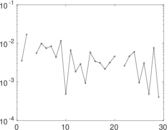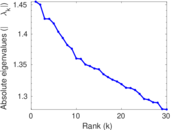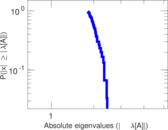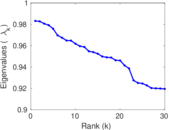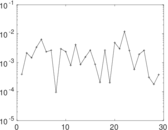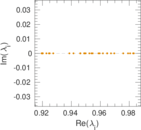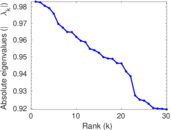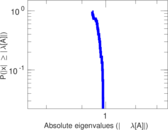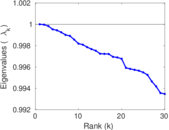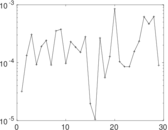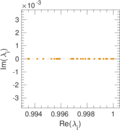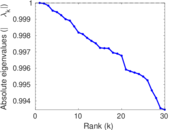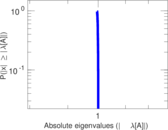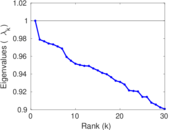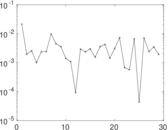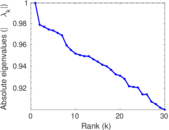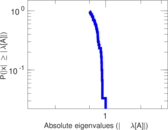This network consists of the wikilinks of the Wikipedia in the Simple English language (simple). Nodes are Wikipedia articles, and directed edges are wikilinks, i.e., hyperlinks within one wiki. In the wiki source, these are indicated with [[double brackets]]. Only pages in the article namespace are included.

 Code `Wsimple` Internal name `wikipedia_link_simple` Name Wikipedia links (simple) Data source http://dumps.wikimedia.org/ AvailabilityDataset is available for download Consistency checkDataset passed all tests Category Hyperlink network Node meaning Article Edge meaning Wikilink Network format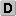Unipartite, directed Edge typeUnweighted, no multiple edges ReciprocalContains reciprocal edges Directed cyclesContains directed cycles Loops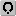Contains loops

## Statistics

 Size n = 185,262 Volume m = 5,098,259 Loop count l = 513 Wedge count s = 1,999,105,088 Claw count z = 3,534,911,521,252 Triangle count t = 197,848,138 Square count q = 77,830,596,597 4-Tour count T4 = 630,648,863,542 Maximum degree dmax = 18,962 Maximum outdegree d+max = 2,039 Maximum indegree d−max = 18,446 Average degree d = 55.038 4 Size of LCC N = 185,198 Size of LSCC Ns = 140,912 Relative size of LSCC Nrs = 0.760 609 Diameter δ = 10 50-Percentile effective diameter δ0.5 = 2.934 41 90-Percentile effective diameter δ0.9 = 3.932 31 Median distance δM = 3 Mean distance δm = 3.494 77 Gini coefficient G = 0.790 403 Balanced inequality ratio P = 0.180 973 Outdegree balanced inequality ratio P+ = 0.194 384 Indegree balanced inequality ratio P− = 0.163 220 Tail power law exponent γt = 1.891 00 Tail power law exponent with p γ3 = 1.891 00 p-value p = 0.000 00 Outdegree tail power law exponent with p γ3,o = 1.961 00 Outdegree p-value po = 0.000 00 Indegree tail power law exponent with p γ3,i = 1.621 00 Indegree p-value pi = 0.000 00 Degree assortativity ρ = −0.047 422 8 Degree assortativity p-value pρ = 0.000 00 In/outdegree correlation ρ± = +0.637 793 Clustering coefficient c = 0.296 905 Directed clustering coefficient c± = 0.783 781 Operator 2-norm ν = 700.682 Cyclic eigenvalue π = 682.022 Spectral separation |λ1[A] / λ2[A]| = 1.529 10 Reciprocity y = 0.502 593 Non-bipartivity bA = 0.851 060 Normalized non-bipartivity bN = 0.052 780 8 Algebraic non-bipartivity χ = 0.101 359 Spectral bipartite frustration bK = 0.000 611 736 Controllability C = 68,664 Relative controllability Cr = 0.370 632

## Plots

### Degree distribution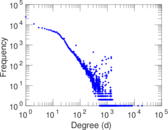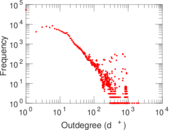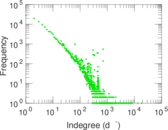### Cumulative degree distribution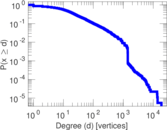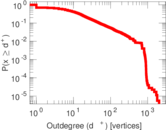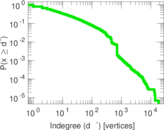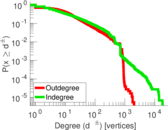### Lorenz curve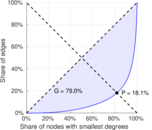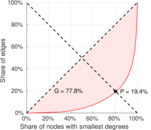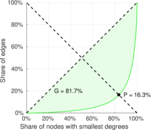### Degree assortativity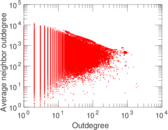### Zipf plot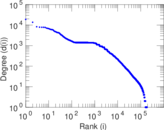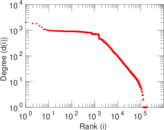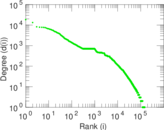### Hop distribution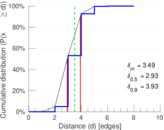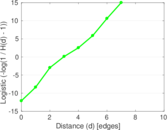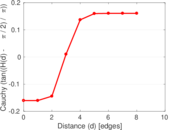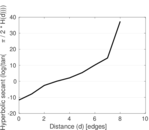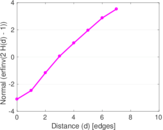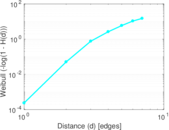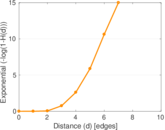### In/outdegree scatter plot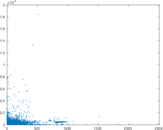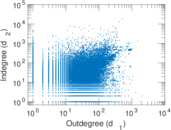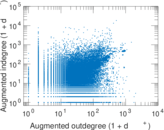### Average neighbor degree distribution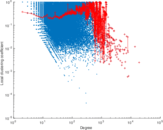### SynGraphy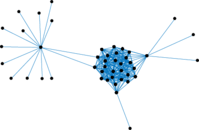### Matrix decompositions plots